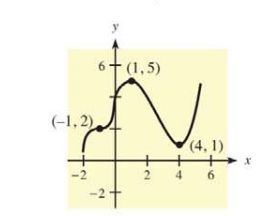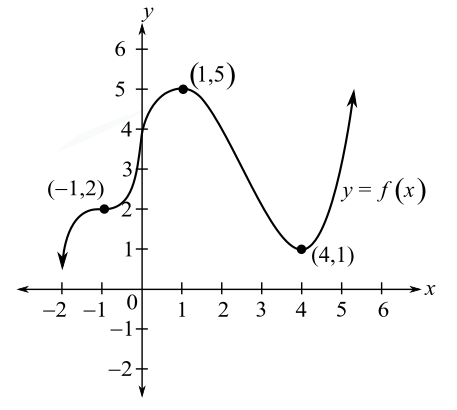Chapter 10.1, Problem 1E### Mathematical Applications for the ...

11th Edition
Ronald J. Harshbarger + 1 other
ISBN: 9781305108042

#### Solutions

Chapter
Section### Mathematical Applications for the ...

11th Edition
Ronald J. Harshbarger + 1 other
ISBN: 9781305108042
Textbook Problem

# In Problems 1 and 2, use the indicated points on the graph of y = f ( x ) to identify points at which f(x) has (a) a relative maximum, (b) a relative minimum, and (c) a horizontal point of inflection.(a)

To determine

The point(s) indicating the relative maximum by the use of the indicated points on the graph of y=f(x) as follows:Explanation

Given Information:

The provided figure of the graph of y=f(x):

Explanation:

The relative maximum can be evaluated as follows by two ways, it can be understood as reaching a peak after increasing, and then going for a downfall.

Consider the given graph:

(b)

To determine

The point(s) indicating the relative minimum by the use of the indicated points on the graph of y=f(x) as follows:(c)

To determine

The point(s) indicating the horizontal point of inflection by the use of the indicated points on the graph of y=f(x) as follows:### Still sussing out bartleby?

Check out a sample textbook solution.

See a sample solution

#### The Solution to Your Study Problems

Bartleby provides explanations to thousands of textbook problems written by our experts, many with advanced degrees!

Get Started

#### In Exercises 2340, find the indicated limit. 25. limx3x

Applied Calculus for the Managerial, Life, and Social Sciences: A Brief Approach

#### Determine whether f'(0) exists. f(x)={xsin1xifx00ifx=0

Single Variable Calculus: Early Transcendentals, Volume I

#### Define the validity of measurement and explain why and how it is measured.

Research Methods for the Behavioral Sciences (MindTap Course List)